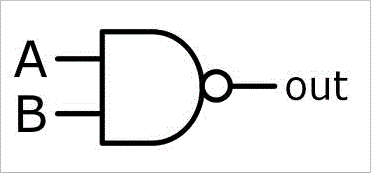top of page

# Logical operations

Computers represent all data as 1's and 0's and are therefore binary devices. Boolean algebra looks at data that, when evaluated results in either true or false. Propositional logic is about following mathematical rules to simplify logical statements. This topic covers:

• Truth tables for Boolean expressions

• Encryption

• Applying logical operations, such as masking and clearing registers

• De Morgan’s laws

• Simplifying Boolean expressions

## NANDGate – The opposite of an AND gate. Gives a false output (0) if all inputs are true, otherwise gives an output of true (1).

Logic Gates# Applying Logical Operations

### ​

This is used in order to determine the value of a bit stored in memory. This is done by applying an AND logical operator to the data with a mask. For example, if we waned to get the 4th bit in 8-bit number 10111100, then you would apply a mask with all digits being 0, except in the value you are checking for. Therefore, you would use 00010000 along with the AND operator. The output will only be a 1 if the bit you’re looking for is also a 1. Else, a 0 will be the output, as both values must be true for an AND gate to give an output of 1.

### Clearing Registers

A mask is also used here, with an AND gate being used too. To clear a register, all bits must be 0. Therefore, a mask of all 0’s is applied, as any value the register holds when used with a 0 will always give a value of 0, as both values will never be 1.

Another method is to use an XOR gate with the exact same data within the register. AN XOR gate will always give a value of 0 if both values entered are a 1, hence why this works.

## ​

### Encryption

This is used to convert data into cipher text that makes it virtually impossible for a human to understand the text. In order to decrypt the text, a decryption key is required that is usually only shared with the sender and receiver. To encrypt data, the key is applied to the data using an XOR gate and decrypted the same way.

Applying

## ​

### De Morgan’s Law

De Morgan was a man who introduced laws into propositional logic. His law stated that Not (A and B) is the same as Not A or Not B. To put it simply, when separating out an entire testament with a NOT gate, it works a bit like maths; with the Not(A) becoming NOTA, like a multiplication. The only thing that changes is the operator. A NOT gate will always change an AND to an Or and an OR to an AND.

### Tautologies

A tautology is when a proposition end in the same result, regardless of any values entered. For example, (NOTA) OR (A) would simply give the output of whatever value was in A. This is used to simplify down larger expressions. For example, in here the testament would be replaced with A.

Simp
bottom of page